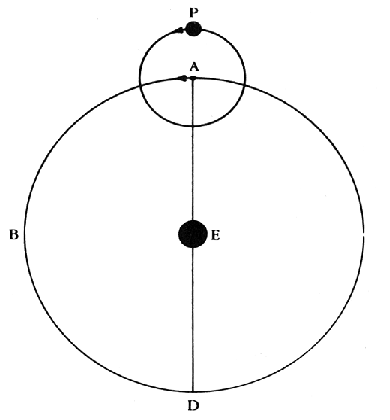The small circle centered around Point A at the top of the figure is an example of an epicycle. The idea was, the center of the epicycle (Point A) would rotate uniformly around the Earth (Object E), while the planet (Object P) would rotate uniformly around Point A. The combination of these two motions could produce an apparent retrograde motion as seen from the Earth. (Imagine Object P on the bottom of the epicycle, where it would be moving opposite to the rotation of Point A.) These geometrical constructions were used because early astronomers were convinced that only uniform circular motion was appropriate for describing the paths of heavenly bodies. But simple circular models could not explain the odd, looping motions of the planets, so they turned to more elaborate models which piled circles upon circles.